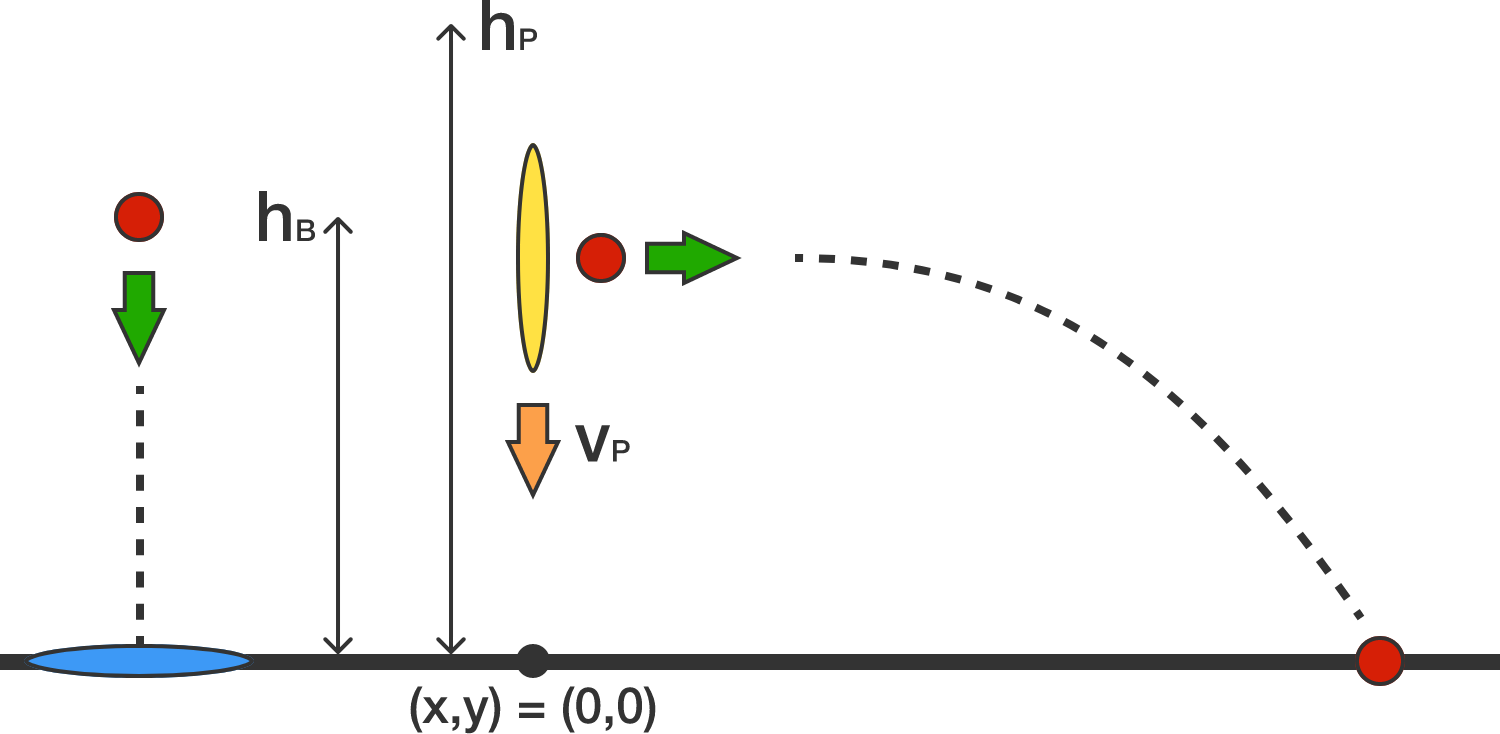# Portal PhysicsThe image above shows two circular planar portals: a blue portal, with its axis oriented in the vertical direction, and an orange portal, with its axis oriented in the horizontal direction. The blue portal is situated at $y=0$, and its $x$-coordinate is irrelevant. The orange portal is situated at $x=0$, and its height ($y$-position) varies as described later.

The rules of portal physics are the following:

• An object enters one portal and emerges from the other
• Portals do not conserve vector momentum or total energy (kinetic + potential)
• Portals do conserve the scalar speed of objects that enter / exit them
• An object entering one portal at its center on a trajectory perpendicular to its axis, will emerge from the other portal in like manner
• Portals do not impart their own velocity to objects which pass through them
• Aside from their own strange properties, portals do not otherwise alter the physics of nearby objects and environments
• It takes zero time to go through a pair of portals

At time $t = 0$, two things happen simultaneously:

• A massive ball drops from its initial resting position at height $h_B$ and falls under the influence of a uniform downward gravitational acceleration $g$. It falls toward the center of the blue portal.

• The orange portal (position defined by its center) begins to descend from its initial vertical position $h_P$ at a constant speed $v_P$.

Consider the $x$-coordinate of the ball at the instant at which it intersects the $x$-axis after emerging from the orange portal. The value of $h_B$ which maximizes this quantity can expressed as:

${h_{B_\text{max}} = \frac{a}{b} g \left(\frac{h_P}{v_P}\right)^2}.$

If $a$ and $b$ are coprime positive integers, determine the value of $a+b$.

×